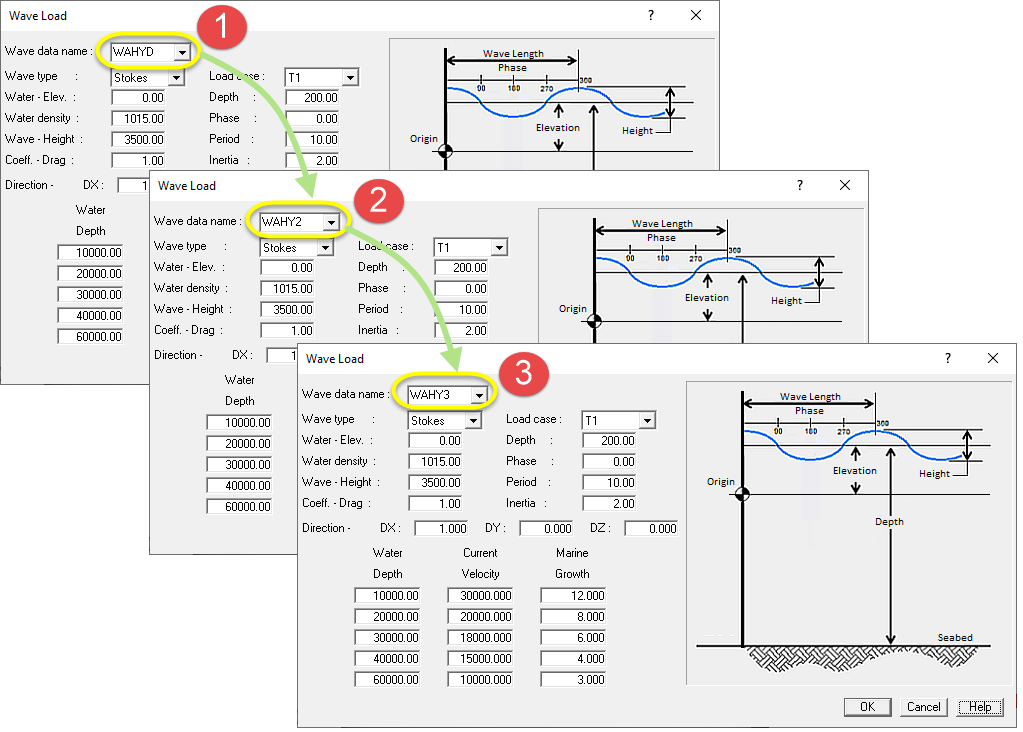# Waves and Sea Currents

### Waves

Calculate the effect of marine waves on fully or partially submerged piping systems.

AutoPIPE designs your wave load in great detail, including various types of wave shapes, allowing you to configure the elevation, depth, phase and direction of propagation. Adjust even the growth of marine deposits.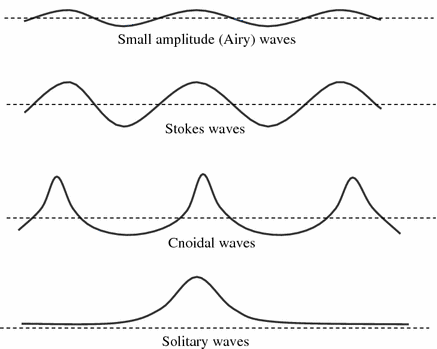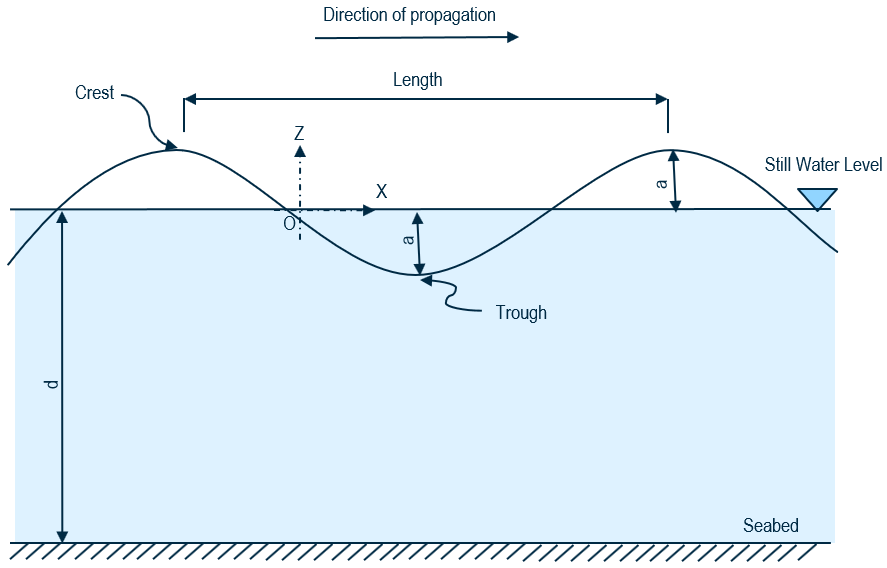AutoPIPE includes the following wave load theories:

• Airy wave theory (first order lineal).
• Stokes wave theory (up to fifth order).
• Stream function wave theory – near breaking wave criteria.
• CCurrent (no wave theory).
• LBuoyancy effects are not included
• Marine growth and current velocity can be entered at different dept.

### Hydrodynamic Forces

• Airy wave theory – small amplitude.
• Stokes wave theory – intermediate and deep water.
• Stream function wave theory – near breaking wave criteria.
• Hydrodynamic forces:
• Inertia forces $$F_I = C_m \times \rho \times V \times \vec{u}$$
• Drag forces $$F_D = \frac{1}{2} C_d \times \rho \times A \times u \times | u |$$
• Lift forces $$F_L = \frac{1}{2} C_L \times \rho \times A \times u \times | u |$$
>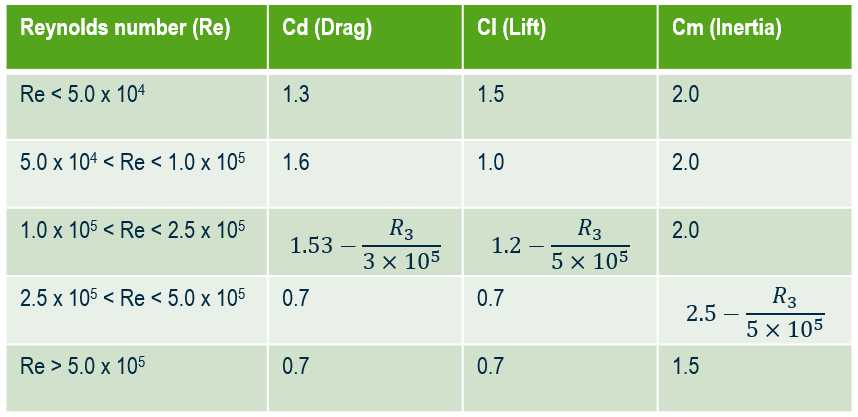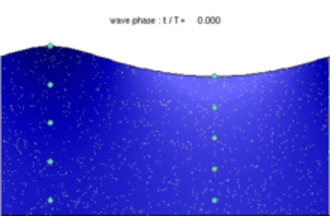How is the data entered?

• Use Xtra Data > Hydrodynamic Data to insert user defined coefficients.
• Suggested values for cylindrical submerged bodies based on Reynold’s number.
• Provide relatively close spacing in areas where wave tough and crest are expected.
• Evaluation of wave loading may should be performed at different phase angles (from 0 to 180 degrees).
• Motion of particles during wave passage (closed loop for Airy).

To study the incidence of waves / current on a pipe, it must be analyzed in its entire circumference. In AutoPIPE you can duplicate any wave / current load and change the direction to have your full range of loads in no time.

### Hydrodynamic User Factors

You can modify the mass coefficient, drag coefficient and lift coefficient of any element by inserting user hydrodynamic factors.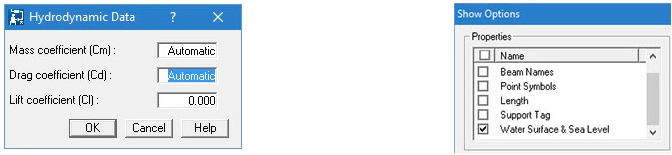### Data Duplication